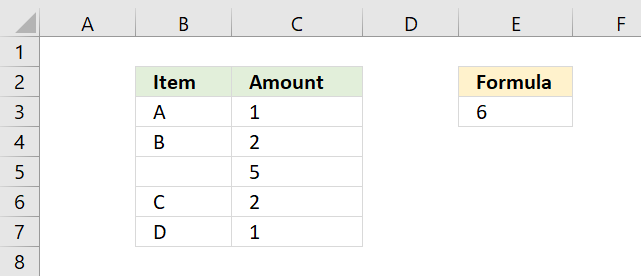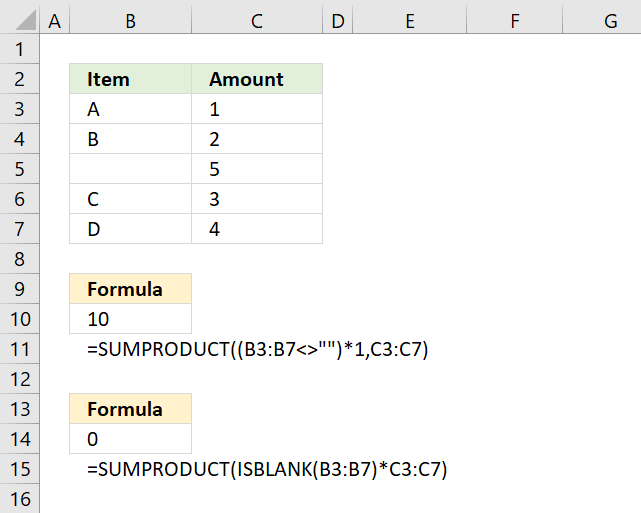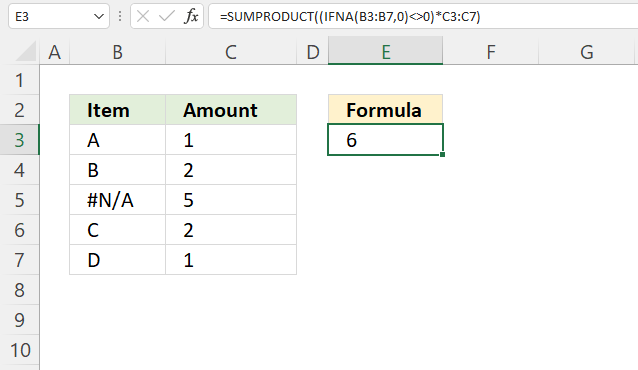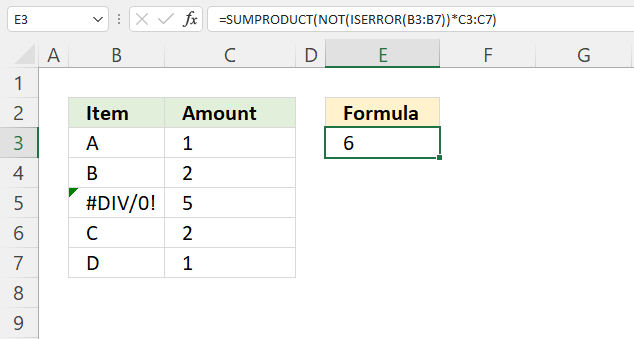Author: Oscar Cronquist Article last updated on January 08, 2023This article describes ways to work with the SUMPRODUCT function and blanks, error values, N/A# errors.

## 1. SUMPRODUCT if not blankThe above image demonstrates how to ignore blank cells in a SUMPRODUCT formula. The following formula is shown in cell E3.

=SUMPRODUCT(NOT(ISBLANK(B3:B7))*C3:C7)

It adds numbers and returns a total if the corresponding value in B3:B7 is not a blank cell. For example, cells B3, B4, B6, and B7 have values and the corresponding cells in C3:C7 are C3, C4, C6, and C7. The total is 1 + 2 + 2 + 1 equals 6.

There is no need for an IF function, simply use the ISBLANK function and then multiply with the corresponding cell range.

### 1.1 Explaining formula in cell E3

#### Step 1 - Identify blank cells

The ISBLANK function returns TRUE or FALSE based on if a cell is blank or not. Since we are using a cell range the ISBLANK function returns an array with the same size as the cell range.

ISBLANK(value)

ISBLANK(B3:B7)

becomes

ISBLANK({"A";"B";"";"C";"D"})

and returns

{FALSE; FALSE; TRUE; FALSE; FALSE}

#### Step 2 - Convert boolean to their opposites

The NOT function comes in handy when you want to convert the boolean values to their opposites. For example, TRUE becomes FALSE and FALSE becomes TRUE.

NOT(ISBLANK(B3:B7))

becomes

NOT({FALSE; FALSE; TRUE; FALSE; FALSE})

and returns {TRUE;TRUE;FALSE;TRUE;TRUE}.

#### Step 3 - Multiply with numbers

The next step is to multiply the boolean array with cell range C3:C7. We can do that by using the asterisk character.

TRUE * number = number
FALSE * number = 0 (zero)

NOT(ISBLANK(B3:B7))*C3:C7

becomes

{TRUE; TRUE; FALSE; TRUE; TRUE}*C3:C7

becomes

{TRUE; TRUE; FALSE; TRUE; TRUE}*{1; 2; 5; 2; 1}

and returns {1; 2; 0; 2; 1}.

The SUMPRODUCT function then adds all numerical values in the array returning 6 in cell E3.

SUMPRODUCT(NOT(ISBLANK(B3:B7))*C3:C7)

becomes

SUMPRODUCT({1; 2; 0; 2; 1})

and returns 6. 1+2+0+2+1 = 6

## 2. SUMPRODUCT returns nothing (blank)Cell range B3:B7 contains a formula that sometimes returns a character and sometimes a blank. The ISBLANK function won't work in this case, see cell B14, it returns 0 which is incorrect.

We need to rely on the larger than and smaller than characters <>, see the formula in cell B10.

=SUMPRODUCT((B3:B7<>"")*C3:C7)

Together like this <> means not equal to. Two double quotes "" is nothing.

#### Step 1 - Identify cells returning nothing

B3:B7<>"" is a logical expression and returns an array of boolean values with as many values as the number of cells in the cell range B3:B7.

B3:B7<>""

becomes

{"A"; "B"; ""; "C"; "D"}<>""

and returns {TRUE; TRUE; FALSE; TRUE; TRUE}.

#### Step 2 - Multiply with numbers

The parentheses determine the order of calculations, we need it to compare the cell range with nothing before multiplying with cell range C3:C7.

(B3:B7<>"")*C3:C7

becomes

{TRUE; TRUE; FALSE; TRUE; TRUE}*C3:C7

becomes

{TRUE; TRUE; FALSE; TRUE; TRUE}*{1; 2; 5; 3; 4}

and returns {1; 2; 0; 3; 4}.

#### Step 3 - Add numbers and return a total

The SUMPRODUCT function sums all values in the array.

SUMPRODUCT({1; 2; 0; 3; 4})

and returns 10 in cell B10. 1 + 2 + 0 + 3 + 4 = 10.

## 3. SUMPRODUCT if not NAThe formula in cell E6 adds numbers from C3:C7 if the corresponding values on the same row in B3:B7 are not a N/A# error and returns a total.

Formula in cell E3:

=SUMPRODUCT((IFNA(B3:B7, 0)<>0)*C3:C7)

### Explaining formula in cell E3

#### Step 1 - Identify NA errors

The IFNA function handles #N/A errors only, it returns a specific value if the formula or cell returns a #N/A error.

IFNA(valuevalue_if_na)

IFNA(B3:B7, 0)

becomes

IFNA({"A"; "B"; #N/A; "C"; "D"}, 0)

and returns {"A"; "B"; 0; "C"; "D"}.

Notice how the N/A error value returns a 0 (zero).

#### Step 2 -  Check if value is 0 (zero)

The less than and the greater than character combined evaluates to "not equal to", the result is a boolean value TRUE or FALSE.

IFNA(B3:B7, 0)<>0

becomes

{"A"; "B"; 0; "C"; "D"}<>0

and returns {TRUE; TRUE; FALSE; TRUE; TRUE}.

#### Step 3 - Multiply with numbers

The parentheses let you control the order of operation, the asterisk multiples the array with the numbers in C3:C7.

(IFNA(B3:B7, 0)<>0)*C3:C7

becomes

{TRUE; TRUE; FALSE; TRUE; TRUE}*C3:C7

becomes

{TRUE; TRUE; FALSE; TRUE; TRUE}*{1; 2; 5; 2; 1}

and returns {1; 2; 0; 2; 1}.

SUMPRODUCT((IFNA(B3:B7, 0)<>0)*C3:C7)

becomes

SUMPRODUCT({1; 2; 0; 2; 1})

and returns 6 in cell E3. 1 + 2 + 0 + 2 + 1 equals 6.

## 4. SUMPRODUCT if not errorThis formula ignores all error values and adds only numbers where the corresponding value on the same row is not an error.

Formula in cell E3:

=SUMPRODUCT(NOT(ISERROR(B3:B7))*C3:C7)

For example, cell range B3:B7 contains values except for cell B5. It contains an error value, the formula adds numbers from cells C3, C4, C6, and C7 but not cell C5.

### Explaining formula in cell E3

#### Step 1 - Identify errors

The ISERROR function returns a boolean value TRUE or FALSE if the value is an error value.

ISERROR(value)

ISERROR(B3:B7)

becomes

ISERROR({"A"; "B"; #N/A; "C"; "D"})

and returns {FALSE; FALSE; TRUE; FALSE; FALSE}.

#### Step 2 -  Convert boolean value to their opposites

The NOT function converts boolean values to their opposites. For example, TRUE becomes FALSE and FALSE becomes TRUE.

NOT(value)

NOT(ISERROR(B3:B7))

becomes

NOT({FALSE; FALSE; TRUE; FALSE; FALSE})

and returns {TRUE; TRUE; FALSE; TRUE; TRUE}.

#### Step 3 - Multiply with numbers

The parentheses let you control the order of operation, the asterisk multiples the array with the numbers in C3:C7.

NOT(ISERROR(B3:B7))*C3:C7

becomes

{TRUE; TRUE; FALSE; TRUE; TRUE}*C3:C7

becomes

{TRUE; TRUE; FALSE; TRUE; TRUE}*{1; 2; 5; 2; 1}

and returns {1; 2; 0; 2; 1}

SUMPRODUCT(NOT(ISERROR(B3:B7))*C3:C7)

becomes

SUMPRODUCT({1; 2; 0; 2; 1})

and returns 6. 1 + 2 + 0 + 2 + 1 equals 6.

### Get the Excel fileSUMPRODUCT-If-not-blanks.xlsx